Next: Bump Mapping Up: Implementation Previous: Register Combiners

## Tangent Space

Lighting can be computed in an arbitrary 3D coordinate system as long as all vector parameters involved are oriented with respect to the same coordinate system . This allows one to select the most convenient coordinate system for lighting. Tangent space is just such a local coordinate system. There are two reasons why lighting in tangent space is very efficient. The first one is that the normal vector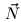equals always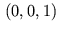in tangent space, thereforeneeds no longer interpolated and normalized. The second reason is that in tangent space the perturbed normal for bump mapping can be read directly from a texture2 as Kilgard develop. The orthonormal basis for tangent space is formed by the surface normal, the surface tangent vector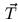, and the binormal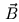defined as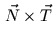(Figure 6)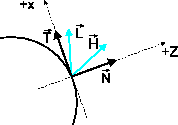For the illumination calculation to proceed properly, the light and half-angle vectors are transformed into tangent space via a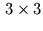matrix whose columns are,, and. The transformations of the light and half-angle vectors should be performed at every pixel; however, if the change of the local tangent space across a polygon is small, a good approximation can be obtained by transforming the vectors only at the polygon vertices. They are then interpolated and normalized in the polygon interior. Therefore tangent space is constructed efficiently on a per-vertex basis with the help of a vertex shader (Section 3.1).Next: Bump Mapping Up: Implementation Previous: Register Combiners
Gerald Schröcker 2002-03-21